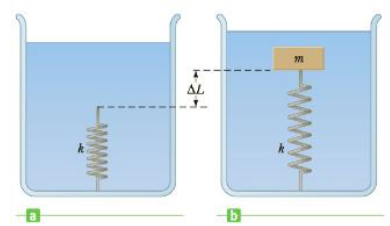Chapter 9, Problem 28P

Chapter
Section
Textbook Problem

A light spring of force constant k = 160 N/m rests vertically on the bottom of a large beaker of water (Fig. P9.28a). A 500-kg block of wood (density = 650 kg/m3) is connected to the spring, and the block–spring system is allowed to come to static equilibrium (Fig. P9.28b). What is the elongation ΔL, of the spring?Figure P9.28

To determine
The elongation ΔL of the spring.

Explanation
At static equilibrium, the sum of forces act on the block of wood is Fy=0BFspingmg=0 . Now, this expression is rearranged for the spring force as Fspring=Bmg=[(ρwaterVblock)m]g=[(ρwater(m/ρwood))m]g . The elongation of the spring is calculated from the definition of restoring force as shown here Fspring=kΔLΔL=Fspring/k .

Given info: Density of water is 103kg/m3 , density of wood is 650kg/m3 , mass of the block is 5.00kg , the force constant of the spring is 160N/m , and acceleration due to gravity is 9.80m/s2 .

The formula for the elongation of the spring is,

ΔL=[ρwater/ρwood1]mgk

• ρwater is density of water

Still sussing out bartleby?

Check out a sample textbook solution.

See a sample solution

The Solution to Your Study Problems

Bartleby provides explanations to thousands of textbook problems written by our experts, many with advanced degrees!

Get Started

Vitamin C deficiency symptoms include: red spots. loose teeth. anemia. all of the above.

Nutrition: Concepts and Controversies - Standalone book (MindTap Course List)

What is the difference between the Milky Way and the Milky Way Galaxy?

Horizons: Exploring the Universe (MindTap Course List)

Define the following terms: a. chromosome b. chromatin

Human Heredity: Principles and Issues (MindTap Course List)

What determines the chemical reactivity of an atom?

Biology: The Dynamic Science (MindTap Course List)